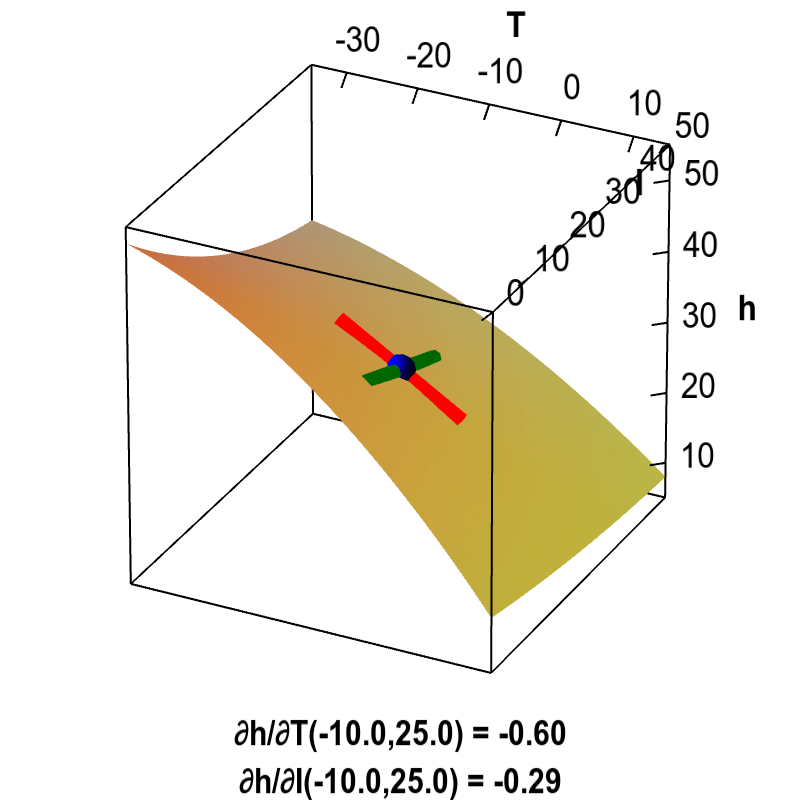# Math Insight

### Applet: Partial derivatives of heating costThe partial derivatives of the heating cost $h(T,I)$ with respect to outside temperature $T$ and amount of insulation $I$ can be viewed as the slopes of the graph in the $T$ direction and in the $I$ direction. The partial derivative $\displaystyle \pdiff{h}{T}$ corresponds to the slope of the red line, and the partial derivative $\displaystyle \pdiff{h}{I}$ corresponds to the slope of the green line. You can drag the blue point around to change the values of $T$ and $I$ where the partial derivatives are calculated.